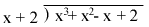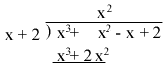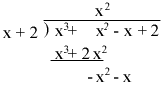Quandaries and Queries How do we divide the following polynomials? (x3 + x2 - x + 2) / (x + 2) Hi Efrat, Set it up as you would to divide integers.x times x2 is x3, hence the quotient starts with x2. Multiplt x + 2 by x2 to getSubtract and bring down the -x.Can you see what to do next? Penny Go to Math Central